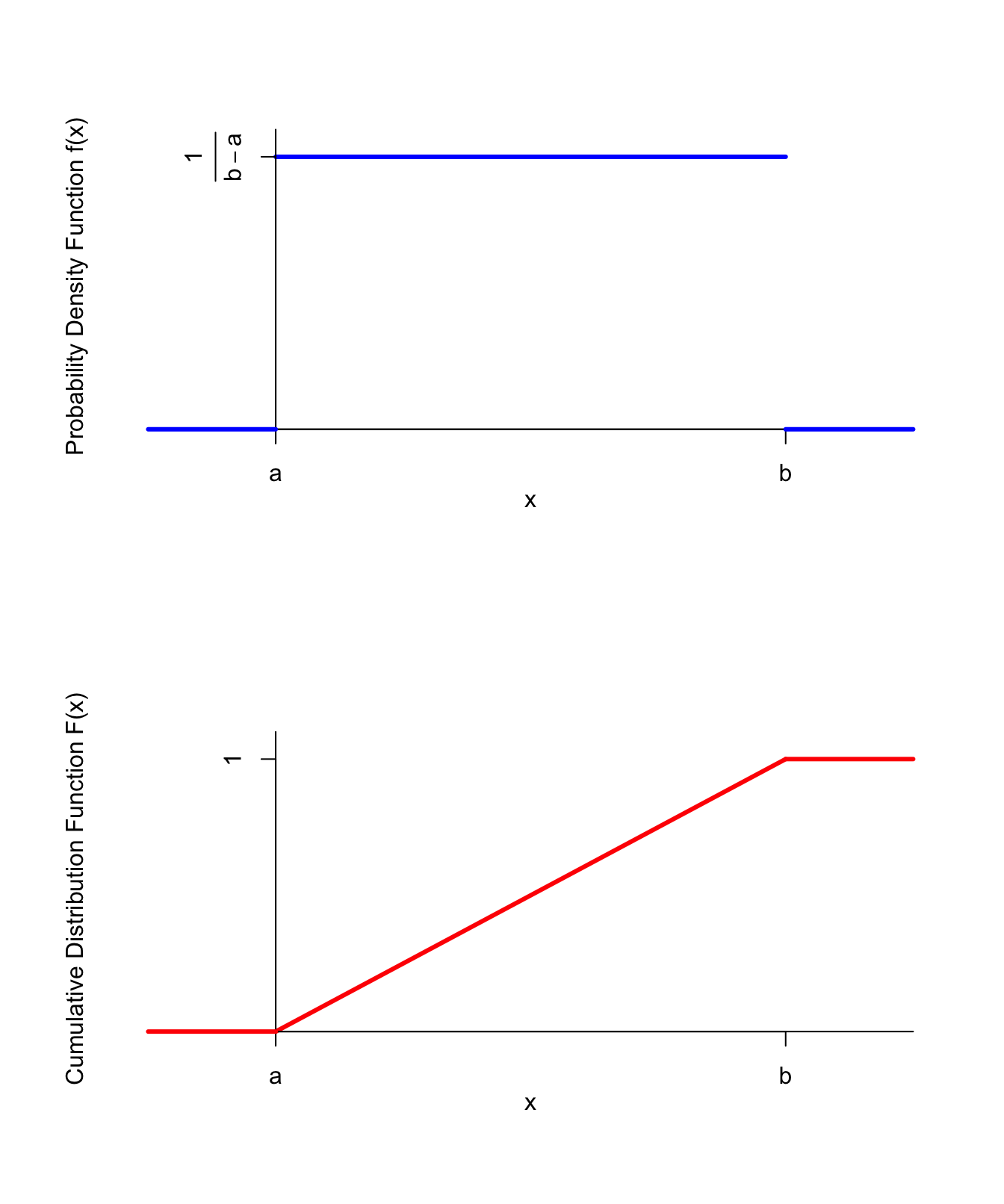# Lesson 34 Uniform Distribution

## Motivation

How do we model a random variable that is equally likely to take on any real number between $$a$$ and $$b$$?

## Theory

Definition 34.1 (Uniform Distribution) A random variable $$X$$ is said to follow a $$\text{Uniform}(a, b)$$ distribution if its p.d.f. is $f(x) = \begin{cases} \frac{1}{b-a} & a \leq x \leq b \\ 0 & \text{otherwise} \end{cases},$ or equivalently, if its c.d.f. is $F(x) = \begin{cases} 0 & x < a \\ \frac{x-a}{b-a} & a \leq x \leq b \\ 1 & x > b \end{cases}.$

The p.d.f. and c.d.f. are graphed below.Figure 34.1: PDF and CDF of the Uniform Distribution

Why is the p.d.f. of a $$\text{Uniform}(a, b)$$ random variable what it is?

• Since we want all values between $$a$$ and $$b$$ to be equally likely, the p.d.f. must be constant between $$a$$ and $$b$$.
• This constant is chosen so that the total area under the p.d.f. (i.e., the total probability) is 1. Since the p.d.f. is a rectangle of width $$b-a$$, the height must be $$\frac{1}{b-a}$$ to make the total area 1.
Example 34.1 You buy fencing for a square enclosure. The length of fencing that you buy is uniformly distributed between 0 and 4 meters. What is the probability that the enclosed area will be larger than $$0.5$$ square meters?
Solution. Let $$L$$ be a $$\text{Uniform}(a=0, b=4)$$ random variable. Note that $$L$$ represents the perimeter of the square enclosure, so $$L/4$$ is the length of a side and the area is $A = \left( \frac{L}{4} \right)^2 = \frac{L^2}{16}.$ We want to calculate $$P(\frac{L^2}{16} > 0.5)$$. To do this, we rearrange the expression inside the probability to isolate $$L$$, at which point we can calculate the probability by integrating the p.d.f. of $$L$$ over the appropriate range. \begin{align*} P(\frac{L^2}{16} > 0.5) &= P(L > \sqrt{8}) \\ &= \int_{\sqrt{8}}^{4} \frac{1}{4 - 0}\,dx \\ &= \frac{4 - \sqrt{8}}{4} \\ &\approx .293. \end{align*}

## Essential Practice

1. A point is chosen uniformly along the length of a stick, and the stick is broken at that point. What is the probability the left segment is more than twice as long as the right segment?

(Hint: Assume the length of the stick is 1. Let $$X$$ be the point at which the stick is broken, and observe that $$X$$ is the length of the left segment.)

2. You inflate a spherical balloon in a single breath. If the volume of air you exhale in a single breath (in cubic inches) is $$\text{Uniform}(a=36\pi, b=288\pi)$$ random variable, what is the probability that the radius of the balloon is less than 5 inches?